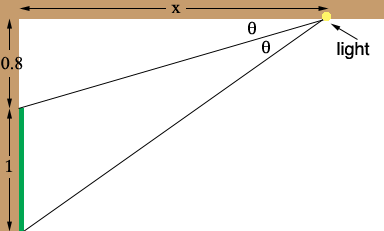SEARCH HOMEMath Central Quandaries & QueriesQuestion from george, a student: The top of a picture 1m high 0.8m from the ceiling. At a point on the ceiling directly in front of the picture, we wish to install a light so that the angle subtended by the picture equals to the angle of depression of the top of the picture. How far out from the wall should the light be installed?Hi George,

In the diagram the distances are measured in metres.From the diagram tan(θ) = 0.8/x and tan(2θ) = 1.8/x. Write tan(2θ) in terms of tan(θ) and solve for x.

I hope this helps,
PennyMath Central is supported by the University of Regina and The Pacific Institute for the Mathematical Sciences.